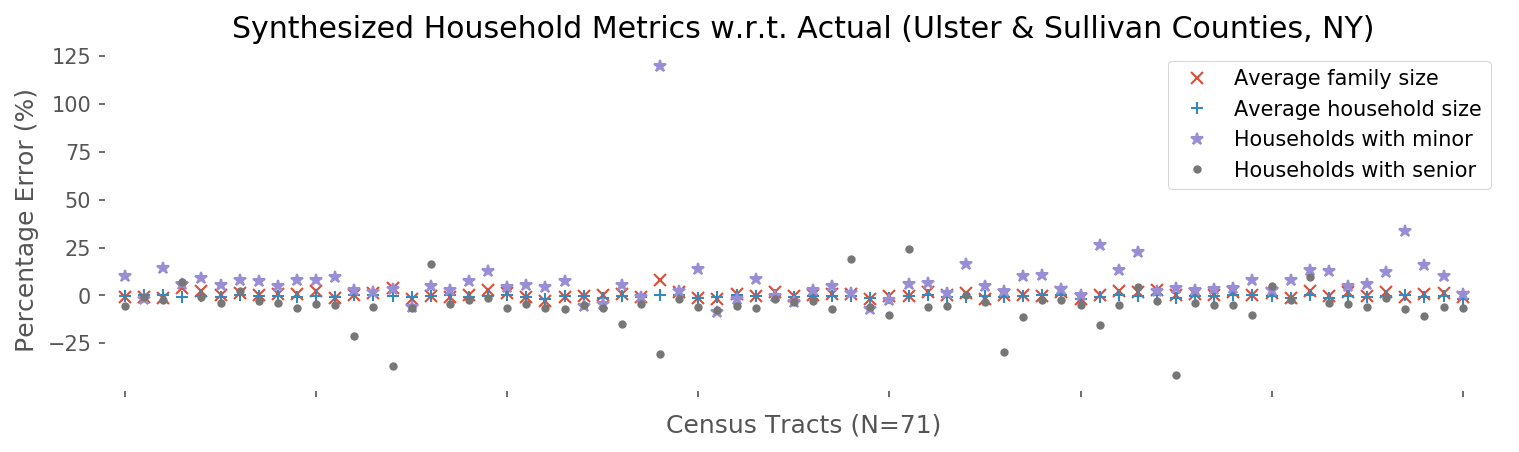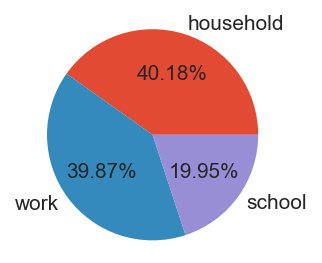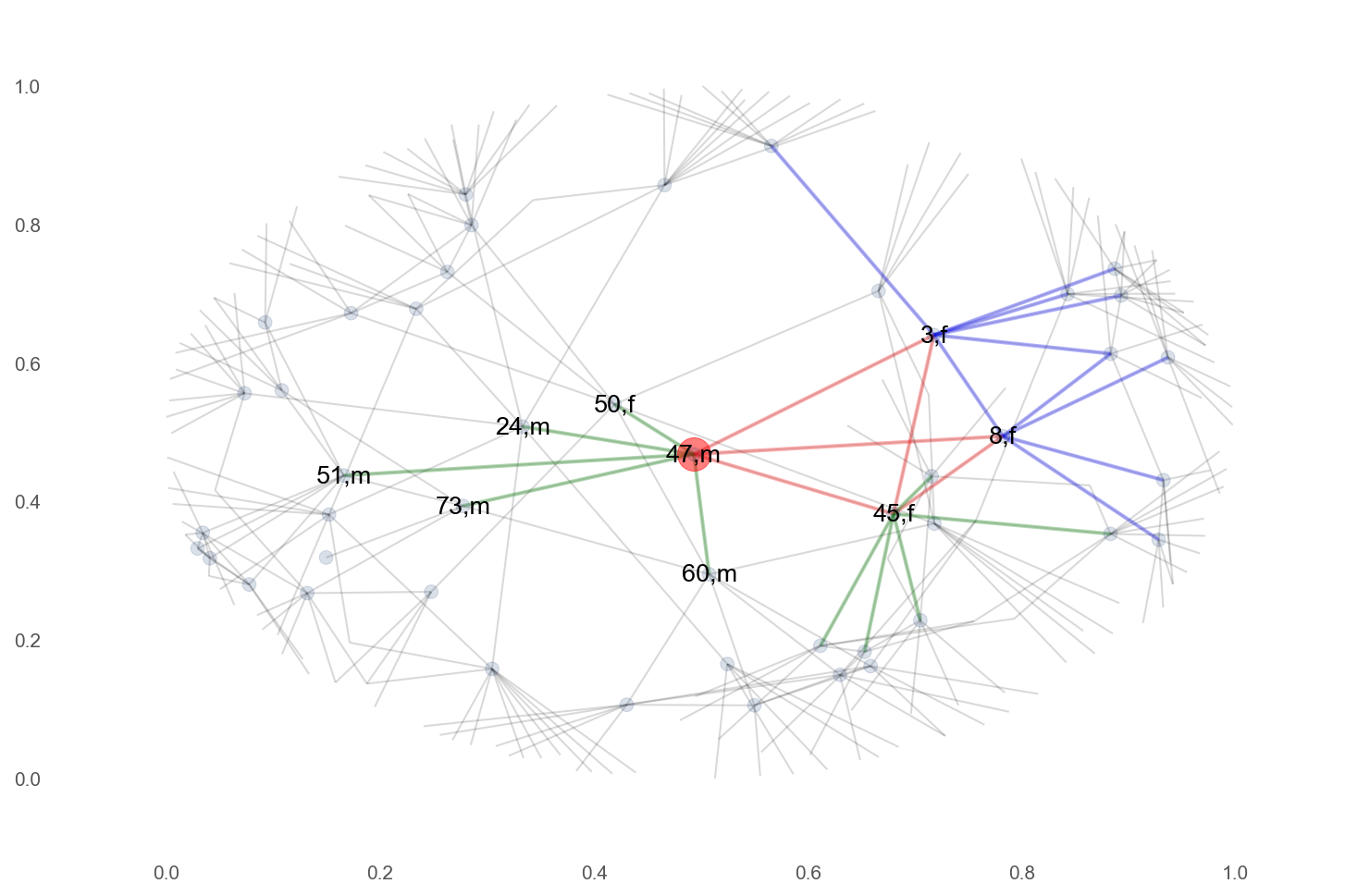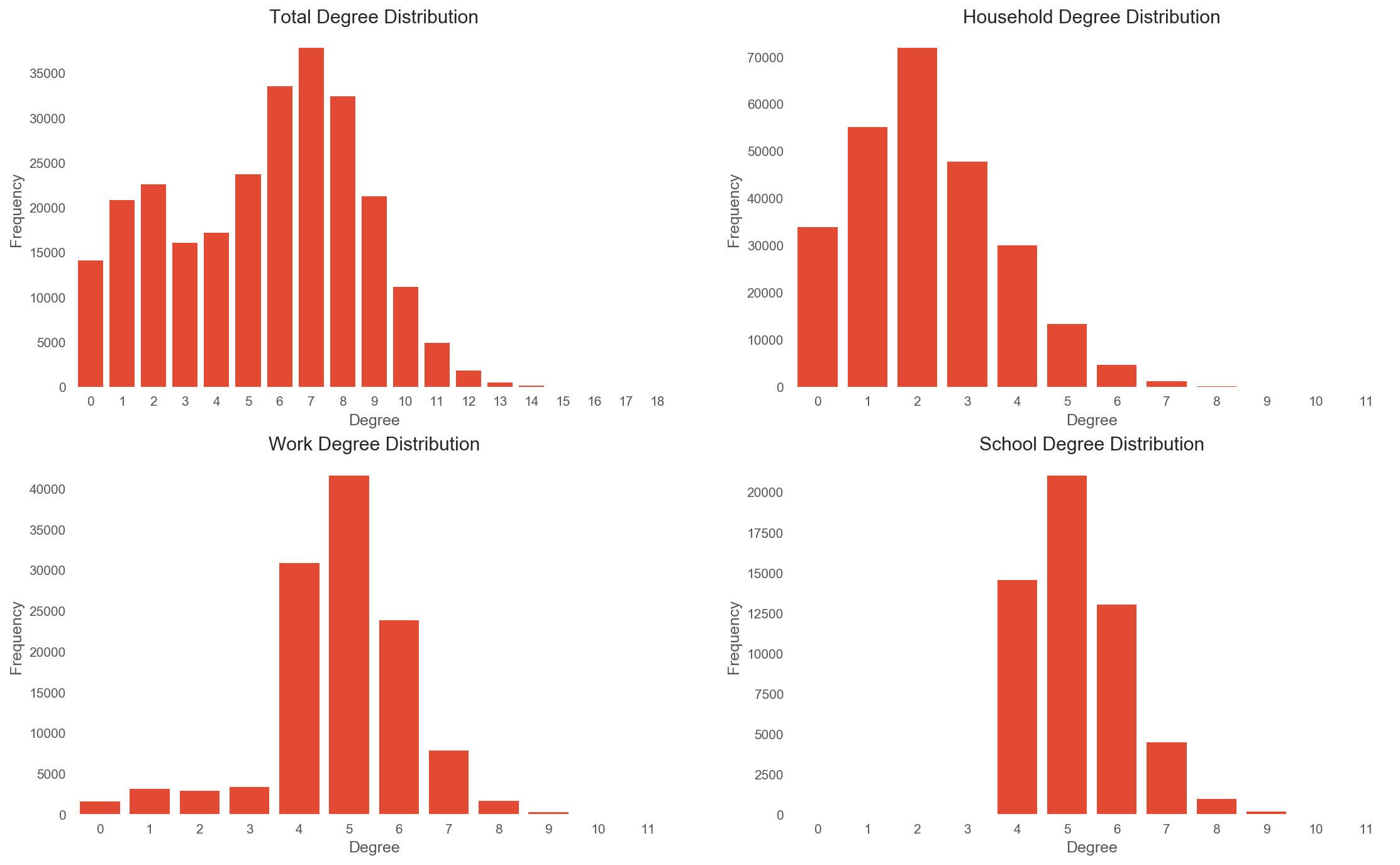# Population Snythesis¶

Notes:

• This notebook is best viewed on nbviewer.
• This work is part of a project conducted in the Center for Social Complexity, and is funded by DTRA.

To simulate "What Happens If a Nuclear Bomb Goes Off in Manhattan?" we first need to create the population (~20 million) living in the area. This notebook shows how we generate our synthetic population on a sample of two counties in New York (Sullivan and Ulster):

• Clean the shapefiles to get a giant connected road network
• Create living spaces
• Houses, work places, and schools
• Create individuals
• Age and sex
• Create relationships
• Households: Families (Husband-wife family, etc.), non-families (Householder living alone, etc.)
• Group quarters (Institutionalized and noninstitutionalized)
• School mate (pre-k, elementary, middle, high school)
• Work colleague (inter-tract and inter-county relations)

## Input (Data Sources)¶

We use five datasets to synthesize our population:

• Demographics: Census-tract level Demographic Profile (DP) TIGER shapefiles (particular shapefile ZIP)
• School info: The Educational Institution dataset
• Establishment numbers: Census Bureau’s County Business Patterns (CBP)
• Workflow: Census Bureau’s Longitudinal Employer- Household Dynamics (LEHD) Origin-Destination Employment Statistics (LODES)

## Output (Deliverables)¶

The output of this notebook is basically a population with six tuples:

individual id, age, sex, social network (a list of ids), household id, workplace/school id

The files in particular are

• population.csv: iid,age,sex,hhid,sid
• space.shp: sid,point,stype (hhold(1-11), school(12), wp(13))
• network.gml: undirected multiplex network (3 types of edges)

# Results (V&V)¶

To verify and validate the synthesized population we examine

• Percentage errors (w.r.t. actual population demographics) at tract level:
1. households with senior/minor
2. average household/family size
• Network statistics for the entire population:
1. average degree, network diameter, clustering coefficient, avg path length
2. comparison of household, school, and work networks
• Map of a census tract:
1. visualization of roads and living spaces

## Percentage Errors¶

In :
with open('errors.pkl', 'rb') as f:

In :
ax = err.plot(style=['x','+','*','.'],figsize=(12,3))
ax.set(xticklabels='',xlabel='Census Tracts (N=71)',ylabel='Percentage Error (%)',#ylim=(-45,45),
xlim=(-1,72),title='Synthesized Household Metrics w.r.t. Actual (Ulster & Sullivan Counties, NY)');## Network Statistics¶

In :
with open('population.pkl','rb') as f:

In :
G = gt.load_graph('k4p3-2.gml')
print('# of nodes: {}\n# of edges: {}'.format(G.num_vertices(),G.num_edges()))
print('Clustering coefficient: {:.2f}'.format(gt.clustering.global_clustering(G)))
print('Pseudo diameter: {}'.format(int(gt.topology.pseudo_diameter(G))))
print('Average degree: {:.2f}'.format(gt.stats.vertex_average(G, 'total')))
counts,bins = gt.stats.distance_histogram(G,samples=1000)
print('Average path length (N=1000): {:.2f}'.format((counts*bins[:-1]).sum()/counts.sum()))

# of nodes: 258672
# of edges: 709213
Clustering coefficient: 0.28
Pseudo diameter: 20
Average degree: 5.48
Average path length (N=1000): 9.17

In :
hhold = gt.load_graph('hhold-2.gml')

hcounts,hbins = gt.stats.vertex_hist(hhold, 'total', float_count=False)
wcounts,wbins = gt.stats.vertex_hist(work, 'total', float_count=False)
scounts,sbins = gt.stats.vertex_hist(school, 'total', float_count=False)

with open('population2.pkl','rb') as f:

print('# of employed:',len(people[(people.age >= 18) & (people.wp.notnull())]))
print('# of students:',len(people[people.age < 18]))

# of employed: 117488
# of students: 54320

In :
counts,bins = gt.stats.vertex_hist(G, 'total')
print('total number of edges: {:.0f}'.format(sum(counts*bins[:-1])))
counts,bins = gt.stats.vertex_hist(hhold, 'total', float_count=False)
print('household edges: {:.0f}'.format(sum(counts*bins[:-1])))
counts,bins = gt.stats.vertex_hist(work, 'total', float_count=False)
print('work edges: {:.0f}'.format(sum(counts*bins[:-1])))
counts,bins = gt.stats.vertex_hist(school, 'total', float_count=False)
print('school edges: {:.0f}'.format(sum(counts*bins[:-1])))

total number of edges: 1418426
household edges: 569912
work edges: 565500
school edges: 283014

In :
labels = ['household','work','school']
sizes = [569912,565500,283014]
fig1, ax1 = plt.subplots(figsize=(2,2))
ax1.pie(sizes, labels=labels, autopct='%1.2f%%')
ax1.axis('equal');In [ ]:
#prepare a 2-hops (FoF) ego network
i0 = '36105952300i550'
#get friends + friends of friends (FoF)
hop2 = set().union(*[[n]+g.neighbors(n) for n in g.neighbors(i0)])
g2 = nx.Graph(g.edges(hop2,data=True))

hholdm = [i0] + [k for k,v in g[i0].items() if v['etype']=='hhold']
hhold_edges = [e for e in g2.edges(hholdm,data=True) if e['etype'] == 'hhold']
work_edges = [e for e in g2.edges(hholdm,data=True) if e['etype'] == 'work']
school_edges = [e for e in g2.edges(hholdm,data=True) if e['etype'] == 'school']

In :
#draw the graph
labels = people.loc[[i0] + g.neighbors(i0)] #[k for k,v in g[i0].items()]
labels = (labels['age'].astype(str)+','+labels['sex']).to_dict()

pos = nx.spring_layout(g2)

fig,ax = plt.subplots();
blues = plt.get_cmap('Blues')
nx.draw_networkx_nodes(g2,pos,nodelist=[i0],alpha=.5)
nx.draw_networkx(g2,pos,nodelist=hop2, node_color=*len(hop2),cmap=blues,vmin=0,vmax=1,alpha=0.15,
node_size=50,font_size=13, labels=labels, ax=ax)

nx.draw_networkx_edges(g2,pos, edge_color='red',edgelist = hhold_edges,width=2,alpha=.3,ax=ax)
nx.draw_networkx_edges(g2,pos, edge_color='blue',edgelist = school_edges,width=2,alpha=.3,ax=ax)
nx.draw_networkx_edges(g2,pos, edge_color='green',edgelist = work_edges,width=2,alpha=.3,ax=ax)
ax.axis('off');In :
f,axes = plt.subplots(nrows=2, ncols=2,figsize=(18,11))

ax = axes[0,0]
counts,bins = gt.stats.vertex_hist(G, 'total')
ax.bar(bins[:-1],counts,tick_label=bins[:-1])
ax.set(xlabel='Degree',ylabel='Frequency',#yscale='log',
title='Total Degree Distribution',xlim=(-0.5,len(counts)-0.5));

ax = axes[0,1]
counts,bins = gt.stats.vertex_hist(hhold, 'total', float_count=False)
counts = G.num_vertices() - sum(counts)
ax.bar(bins[:-1],counts,tick_label=bins[:-1])
ax.set(xlabel='Degree',ylabel='Frequency',#yscale='log',
title='Household Degree Distribution',xlim=(-0.5,len(counts)-0.5));

ax = axes[1,0]
counts,bins = gt.stats.vertex_hist(work, 'total', float_count=False)
counts = len(people[(people.age >= 18) & (people.wp.notnull())]) - sum(counts)
ax.bar(bins[:-1],counts,tick_label=bins[:-1])
ax.set(xlabel='Degree',ylabel='Frequency',#yscale='log',
title='Work Degree Distribution',xlim=(-0.5,len(counts)-0.5));

ax = axes[1,1]
counts,bins = gt.stats.vertex_hist(school, 'total', float_count=False)
counts = len(people[people.age < 18]) - sum(counts)
ax.bar(bins[:-1],counts,tick_label=bins[:-1])
ax.set(xlabel='Degree',ylabel='Frequency',#yscale='log',
title='School Degree Distribution',xlim=(-0.5,len(counts)-0.5));## A Sample Map¶

In :
with open('wps2.pkl', 'rb') as f:

In :
tract = dp.iloc[-5]
#tract houses and wps
thouses = gpd.GeoSeries(people[people.hhold.str.startswith(tract.name)].geometry)
twps = gpd.GeoSeries(wps[wps.index.str.startswith(tract.name)])

#tract
ax = gpd.GeoDataFrame(tract).T.plot(ls='--',color='white') #draw tract borders
markers = {}

ax = thouses.plot(ax=ax,markersize=3,color='orange',linestyle='None') #draw houses
markers['house'] = ax.get_lines()[-1]

ax = twps.plot(ax=ax,markersize=4,color='purple',linestyle='None') #draw workplaces
markers['workplace'] = ax.get_lines()[-1]

edu = school[school.intersects(tract.geometry)] #schools and daycares
ax = edu.plot(ax=ax,markersize=5,color='red',linestyle='None') #draw educational inst
markers['school'] = ax.get_lines()[-1]

ax.axis('off')